# How to Subtract in Excel — Basic Excel Guide

Posted April 5, 2019 by Sheraz Ali in Microsoft Excel

There are many different methods you can use to subtract in Excel. All of them have their own use. Some are perfect for performing subtraction within cells, while others are more suitable for subtracting an entire range of cells.

Rest assured, in this tutorial, I’ll show you how to subtract in excel in simple and easy steps with visual instructions. This will ensure that all confusions and complications are removed and the process is broken down and simplified for your convenience.

### Subtracting One Cell from Different Cells

In order to subtract one cell from a whole range of cells, you will have to understand how formulas work.

For now, all you have to keep in mind is that formulas in Excel always start with a “=” sign. The rest will be made clear to you as the tutorial proceeds.

1. Open Your Excel Workbook.

2. Input your Data, if you already haven’t.

3. In an empty cell, type an equal (=) sign to start your formula.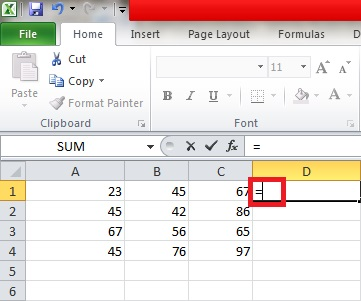4. Type the name of the cell you want to subtract other cells from. In this case, I have chosen to subtract numbers from the cell “A1”.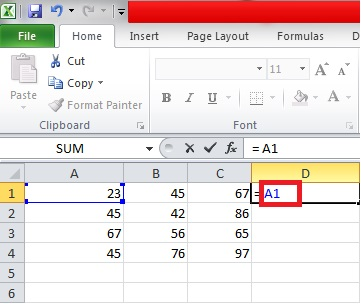5. Type the minus sign (-) followed by the names of the cells you want to subtract the data in “A1” from.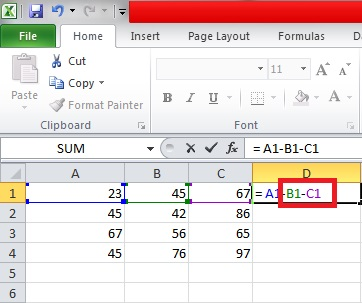6.Hit Enter.

7. The subtracted number will appear in the cell as a result, like this.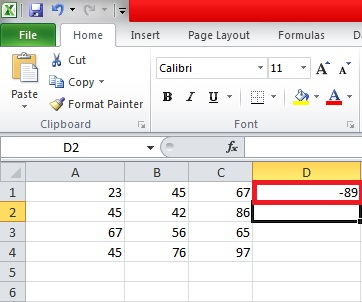### Subtracting Numbers within One Cell

When you’re not working with data from different cells and really just need to subtract a bunch of numbers for the heck of it, here’s how you can do that.

1. Open your Excel Workbook.

2. Click an empty cell.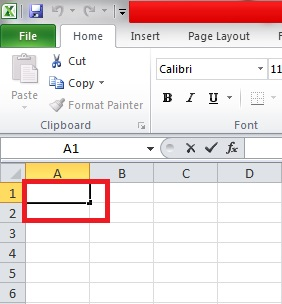3. Start your formula with an equal (=) sign.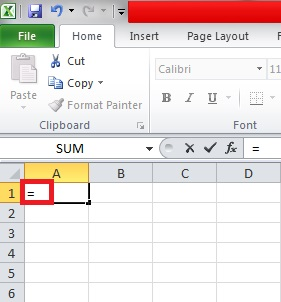4. Type the main number you want to subtract other numbers from. In this case, I have chosen to subtract numbers from 100.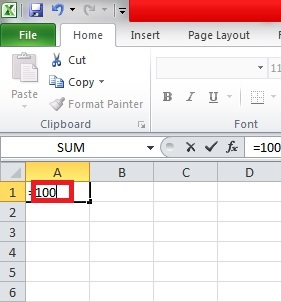5. Now type the numbers that you want to subtract. In this case, I am subtracting 20 and 10 from 100. You can subtract more numbers if you wish. It’s up to you. This is just to give you a general idea.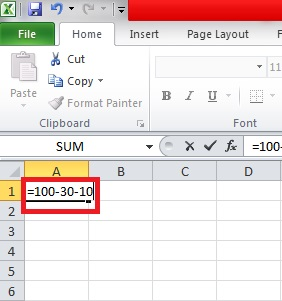6.Hit Enter.

7. The final result will appear in the cell as follows.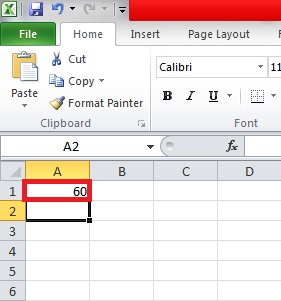### Subtracting an Entire Range of Cells

To subtract a vertical column of cells or an entire range of cells in Excel, here’s what you have to do.

1. Open Your Excel Workbook.

2. Type your main number in the first cell of the column. In this case, I aim to subtract numbers from 5000, so I’ve written it at the very top.3. Now, type all the numbers you want to subtract from 5000 underneath it.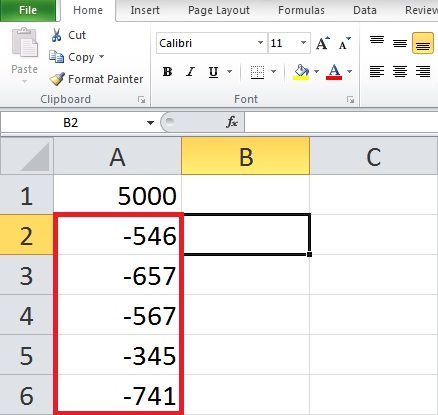4. Click on an empty column. Any empty column will do.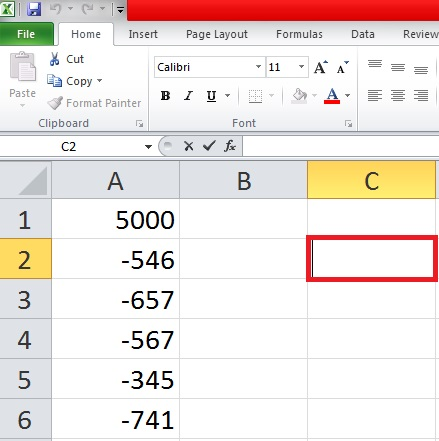5. Start your formula with an equal (=) sign.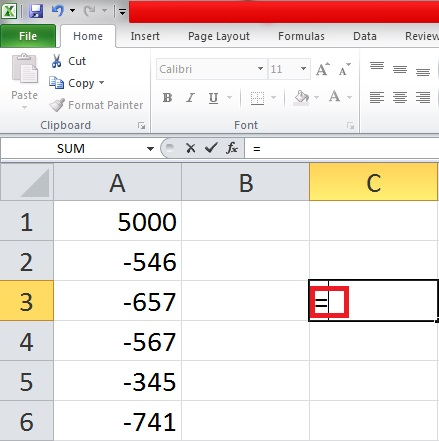6.Type SUM( after the equal sign to insert the SUM formula.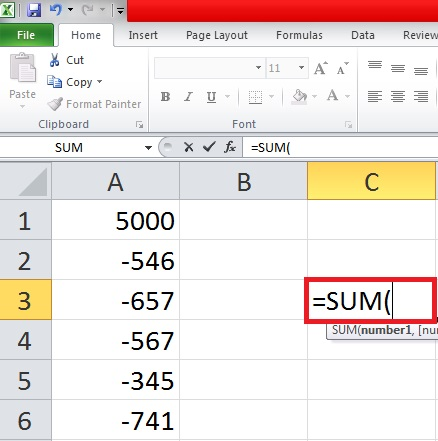7. Now select the entire range of cells or the cells you want to subtract from your main number. This selection must include your main number.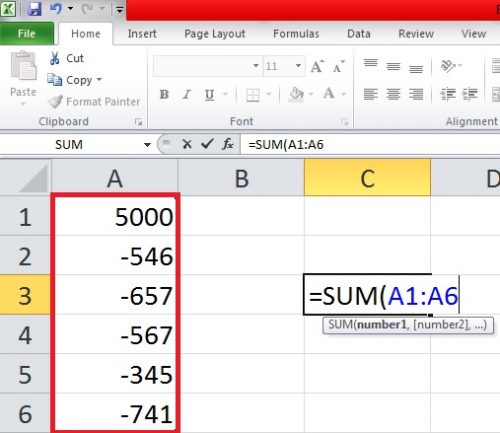8.Hit Enter.

9. The subtracted result will appear as follows.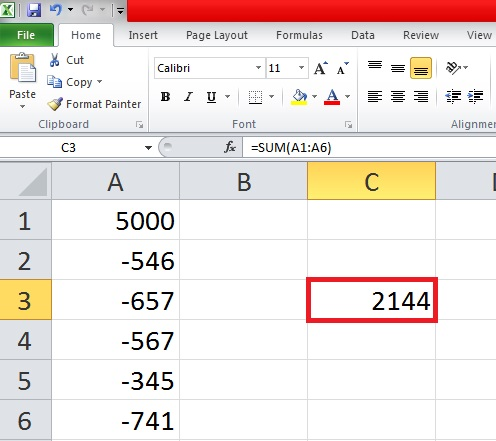This is an easy and fun way to learn how to subtract in excel. If you have confusions or questions, please check these related articles for further clarification.

How to Add in Excel: Easy Methods

If you’re interested in adding a drop-down menu to your excel data, check this tech-recipe out.

How to Add a Drop down list in Excel

### About Sheraz Ali

An established copywriter, with a longstanding experience in a vast array of industries, including but not limited to spirituality, technology, cannabis and travel.
View more articles by Sheraz Ali

#### The Conversation

Follow the reactions below and share your own thoughts.